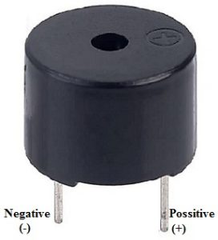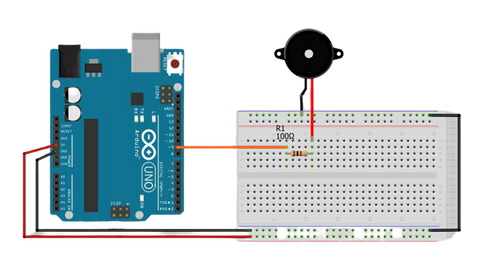# How to use Buzzer with Arduino

We all know that buzzers are the beepers which produce tones. But, do you know what role a Buzzer plays in an Arduino? How is a buzzer used in it? If not, then let's get started!

## What is a Buzzer in Arduino?

An Arduino Buzzer is basically a beeper. The Arduino buzzer is a device that produces sound when an electric current is passed through it. The Arduino buzzer can be directly connected to the Arduino and produce different tones by giving different frequency electric pulses to the buzzer. The Arduino buzzers are most commonly used as beepers in any system, Alarm devices, timers, security systems and to produce sound on confirmation of user input in many systems. The buzzers are of different types such as Mechanical buzzers, Electromechanical buzzers and Piezoelectric buzzers.

The piezoelectric buzzer is most commonly used with the Arduino. As it is lightweight, simple in construction, typically a low-cost product that can generate different sound tones of different frequencies and does not require a separate oscillating circuit.

In this blog, we will produce different sound tones using a buzzer by applying different frequency electric pulses.

## How does the Arduino Buzzer work?

The Positive pin (+ve) of the Arduino buzzer has to be connected to the VCC of Arduino and the Negative pin (-ve) has to be connected to the GND pin of the Arduino.When the Positive pin is connected to the 5 Volts pin of the Arduino directly, the buzzer produces a sound of constant frequency.

When a square wave of a certain frequency (and 50% duty cycle) is applied on the Positive (+ve) pin of the Arduino buzzer generates tones. The different frequencies generate different tones. By changing the frequency on the positive pins of the Arduino buzzer, we can create a melody of the song.

## How to use the Arduino buzzer?

If your buzzer has a sticker on top of it, pull the sticker off. Connect the short pin of the buzzer to the Arduino's ground pin (GND) and the other end to digital pin 9. Using the Arduino, you can make sounds with a buzzer by using different frequency electrical pulses. You have to tell the Arduino on which pin the buzzer is connected, what should be the frequency (in Hertz, Hz) of the electric pulse has to be applied to the buzzer, and for how much time you want it to keep making the tone. So for doing this, we will use the Arduino buzzer tone generation command:

tone(9, 1200, 500);    // tone on pin 9 at 1200 Hz for 1/2 second

The above-mentioned command is the function used to generate an electric signal of a particular frequency and for a particular period of time on a particular pin. So we are using pin number 9 of Arduino to perform such an operation here. When you call the tone() function, you have to pass this function with 3 arguments. I.e, Pin number, frequency of the signal you want to generate, and the time in milliseconds. As per the above-mentioned command, 9 stands for the pin number of the pin you want to use, (Note that the pin you use for this operation should be a PWM pin). The 1200 stands for the frequency you want to generate, and the 500 stands for time in milliseconds, this is the time period that the Arduino generates the frequency for the buzzer.

Also Read - IR Sensor Interfacing with Arduino

Once, the square wave is generated as per what we declared in the tone(); function, to stop the frequency generation noTone(); function has to be used. The syntax for the noTone(); function is mentioned below. If you do not call the noTone(); function, it will keep making a tone until you tell it to stop by calling noTone(pin) or by calling tone() with a different frequency.

noTone(9);   // Stops the generation of a square wave triggered by tone().

For stopping the frequency generation, generated by the tone(); function, the above function has to be called. In the noTone(); an argument with the pin number has to passed, which says the Arduino that to stop the frequency generation on a particular pin. (Note: The same pin has to be used, which was used for the tone generation). So let’s see the complete code and the connection for playing music on the Arduino buzzer.

## Steps to use Buzzer with Arduino

### Step 1: First, grab all the components listed above.### Step 2: Make connections as per the circuit diagram given below:Pin number 9 of Arduino One of the pins of 100 ohms Resistor GND pin of Arduino Negative pin of the buzzer (Shorter pin) Empty 100 ohms resistor pin Positive pin of the buzzer

• Connect the Arduino to the Computer using a USB cable.
• Open, Arduino IDE and select the correct board and port.
• Next, In the top left corner, go to file >> Examples >> 02. Digital >> toneMelody.
• Once the code is opened, upload the code to the Arduino and the melody music sound will be played using the buzzer.

### The below-attached code plays simple jingle-bell music:

``````
/*
* Created by ArduinoGetStarted.com
*
* This example code is in the public domain
*
* Tutorial page:
https://arduinogetstarted.com/tutorials/arduino-piezo-buzzer
*/
#include "pitches.h"
// notes in the melody:int melody[] = {
NOTE_E5, NOTE_E5, NOTE_E5,
NOTE_E5, NOTE_E5, NOTE_E5,
NOTE_E5, NOTE_G5, NOTE_C5, NOTE_D5,
NOTE_E5,
NOTE_F5, NOTE_F5, NOTE_F5, NOTE_F5,
NOTE_F5, NOTE_E5, NOTE_E5, NOTE_E5, NOTE_E5,
NOTE_E5, NOTE_D5, NOTE_D5, NOTE_E5,
NOTE_D5, NOTE_G5};
// note durations: 4 = quarter note, 8 = eighth note, etc,
also called tempo:int noteDurations[] = {
8, 8, 4,
8, 8, 4,
8, 8, 8, 8,
2,
8, 8, 8, 8,
8, 8, 8, 16, 16,
8, 8, 8, 8,
4, 4};
void setup() {
// iterate over the notes of the melody:
int size = sizeof(noteDurations) / sizeof(int);

for (int thisNote = 0; thisNote < size; thisNote++) {

// to calculate the note duration, take one second divided by the
note type.
//e.g. quarter note = 1000 / 4, eighth note = 1000/8, etc.
int noteDuration = 1000 / noteDurations[thisNote];
tone(8, melody[thisNote], noteDuration);

// to distinguish the notes, set a minimum time between them.
// the note's duration + 30% seems to work well:
int pauseBetweenNotes = noteDuration * 1.30;
delay(pauseBetweenNotes);
// stop the tone playing:
noTone(8);
}}
void loop() {
// no need to repeat the melody.}``````

## Conclusion:

The above-written blog gives the knowledge of the Arduino buzzer. How does it work and how to use it with Arduino and to write codes to generate different music sounds.

### 1. How do I make my Arduino beep?

The buzzer can be beeped using a digitalWrite function. By giving the pin number and the state to which the Buzzer is connected on the Arduino.

### 2. What is Active and Passive buzzers:

Active buzzer: An active buzzer has a built-in oscillating source, so it will make sounds when electrified. Can turn it on or off to make various sounds. Only requires an external DC voltage to make it sound (batteries or USB from Arduino).

Passive buzzer: The passive buzzer is an electromagnetic speaker used to generate sound signals of different frequencies. a passive buzzer requires a signal source, which will set the sound signal parameters. An Arduino board can be such a source.

## Related Blogs

### 1. How do I make my Arduino beep?

The buzzer can be beeped using a digitalWrite function. By giving the pin number and the state to which the Buzzer is connected on the Arduino.

### 2. What is Active and Passive buzzers:

Active buzzer: An active buzzer has a built-in oscillating source, so it will make sounds when electrified. Can turn it on or off to make various sounds. Only requires an external DC voltage to make it sound (batteries or USB from Arduino).

Passive buzzer: The passive buzzer is an electromagnetic speaker used to generate sound signals of different frequencies. a passive buzzer requires a signal source, which will set the sound signal parameters. An Arduino board can be such a source.